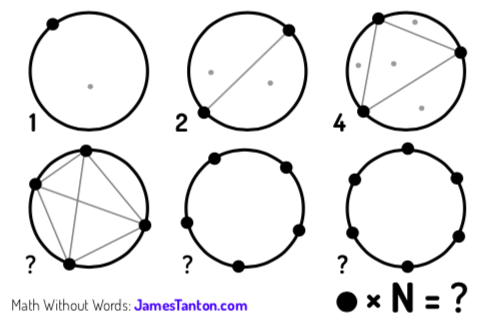On a recent MathsJam Shout, an Old Chestnut appeared (in this form, due to @jamestanton):If you’ve not seen it, stop reading here and have a play with it - it’s a classic puzzle for a reason.

Below the line are spoilers.

### Counting is hard

The first thing you’d probably try is to draw out the lines and count up the regions.

The first circle has 1 region.

The second has 2 regions.

The third has 4. (I’m spreading these out to try to avoid accidental spoilers, by the way.)

The fourth has 8. A pattern seems to be forming.

The fifth has 16. Aha! It must be…

The sixth has 31. Oh. Let’s count them again: nope, definitely 31 rather than 32. What’s going on?

### What is going on?

As you draw more and more points, it gets harder to a: make sure you’re not making your lines coincide and b: count the regions accurately. At MathsJam, we made it to the seventh circle (57 regions) before realising that was about as far as we could go.

So we needed a more methodical approach. The nugget of the solution - for me, at least - was to think about how each line you add to a diagram affects the number of regions - and that’s related to the number of lines it crosses. In fact, adding a line that crosses $n$ other lines adds $n+1$ regions to the circle - which you might like to prove.

So, in adding a fifth point to the fourth circle, we’ll need to add four new lines.

The two lines to the adjacent points each add a single region - they don’t cross any other lines. The lines to the two opposite points each cross two lines, creating three new regions. Overall, that’s 1 + 3 + 3 + 1 new regions, taking the eight regions to 16.

### Let’s go a bit more methodically this time

Going from five to six is a bit trickier ((because counting is hard)).

Let’s consider the five existing points, going anticlockwise from our new point (which I’ll imagine is at the bottom of the circle). Each new line will split the polygon into two parts, which I’ll call ‘left’ and ‘right’.

The first line, to the neighbour, crosses no lines, and adds one region.

The second line, to point 2, splits the circle so there is one point to the left and three to the right, all of which are connected - so this line adds $1 \times 3 + 1$ regions.

The third line splits the circle two on each side, so it adds $2 \times 2 + 1$ regions.

The fourth line mirrors the second, and the fifth line mirrors the first, so I might be tempted to write the number of regions added as:

• $(0\times 4 + 1) + (1 \times 3 + 1) + (2 \times 2 +1) + (3 \times 1 + 1) + (4 \times 0 + 1)=15$

… or better, the new number of regions is $5 + \sum_{i=0}^{4} i(4-i)$

### But we can generalise!

Whenever we add a point to a diagram, the process is going to be identical! Each line will divide the existing points into three sets: those to the left, the one being connected, and those to the right. Every point on the left connects to every point on the right exactly once, so the number of lines cut by the new line is always the number of left points multiplied by the number of right points.

So! If we’re moving from $N-1$ points to $N$, we should be adding:

• $R_n = (N-1) + \sum_{i=0}^{N-2} i( (N-2) - i)$

new regions.

And we can write that explicitly!

• $R_n = (N-1) + (N-2)\sum_{i=0}^{N-2} i - \sum_{i=0}^{N-2} i^2$
• $\dots = (N-1) + (N-2)\frac{(N-2)(N-1)}{2} - \frac{ (N-2)(N-1)(2N-3)}{6}$
• $= \frac{(N-1)}{6} \left[ 6 + 3(N-2)^2 - (N-2)(2N-3) \right]$
• $= \frac{(N-1)}{6} \left[ N^2 - 5N + 12 \right]$

Let’s check that with $N=6$, which we’ve already worked out: $R_n = \frac{5}{6}\left[ 36 - 30 + 12 \right] = 15$ new regions.

How about $N=7$? We’re expecting 26: $R_n=\frac{6}{6}\left[ 49 - 35 + 12\right] = 26$. Hooray!

### Hang on - that’s just the new regions!

So, the number of new regions each time is $\frac{N-1}{6} \left[ N^2 - 5N + 12 \right]$, and we know when there are no points, there is one region.

So, the number of regions altogether is $R_\sum(M) = 1 + \frac{1}{6}\sum_{N=0}^{M} (N-1)(N^2 - 5N + 12)$.

Expanding that and distributing the sum:

$R_\sum(M) = 1 + \frac{1}{6} \sum_{N=0}^{M} N^3 - \sum_{N=0}^{M} N^2 + \frac{17}{6}\sum_{N=0}^{M} N - \sum_{N=0}^{M} 2$

There follows an awful lot of tedious algebra, which ends up giving $R_\sum(M) = \frac{1}{24}\left(M^4 - 6M^3 + 23M^2 - 18M + 24\right)$

Just to check, we know $R_\sum(6)$ is 31: is that $\frac{1}{24}\left( 6^4 - 6\times6^3 + 23\times 6^2 - 18\times 6 + 24 \right)$? The first two terms cancel, and the rest? It’s $\frac{6}{24} \left( 23 \times 6 - 18 + 4\right)$, or $\frac{1}{4} \times 124 = 31$, as required!

Curiously, it turns out that $R_\sum(10) = 256$, which is also a power of 2 (although half as big as it “ought” to be if it followed the doubling pattern.)

• Updated 2021-08-09 to fix LaTeX